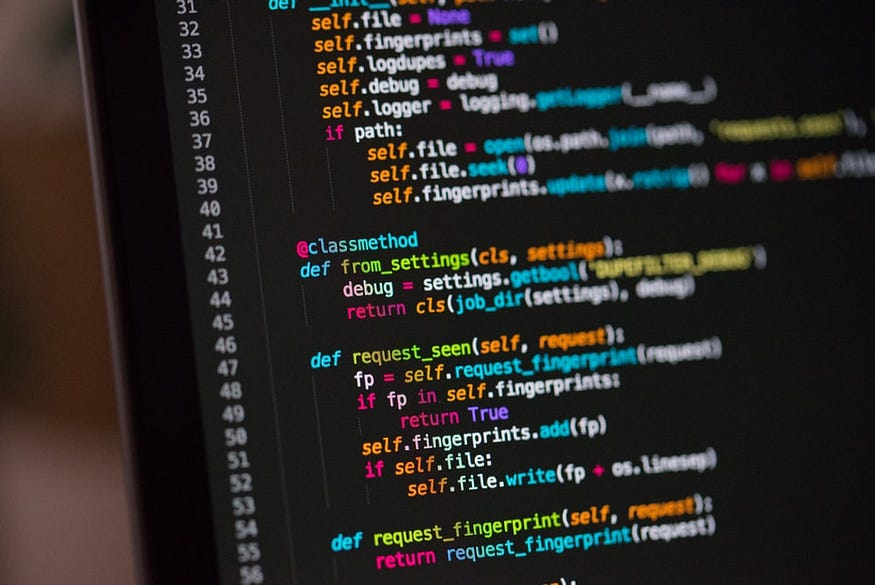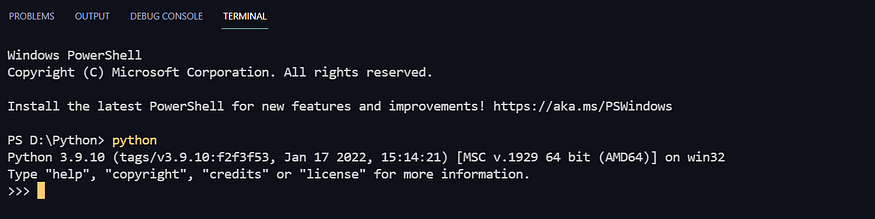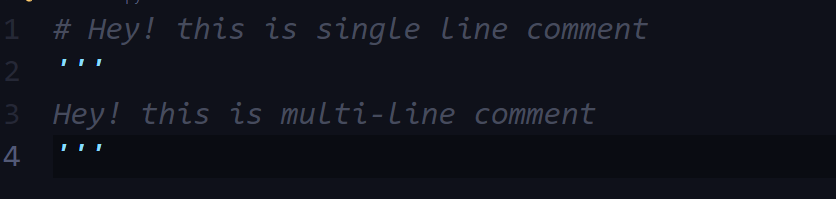# Python: A Quick Revision

## An introduction to Python for beginners

### What is Programming?

To communicate with people, we use one of the comfortable languages similarly when it comes to communicating with computers we use one of the programming languages such as Python, C, C++ and so. A programmer instructs a computer to perform a task through a programming language.

### What is Python?

Python is a high-level programming language. ‘High level’ means it is a programmer-friendly language because its code seems similar to English text and with the help of Python, a programmer instructs a computer.### Features of Python

• Easy to understand
• Open Source
• High-level language
• Portable

Modules: A module is a file containing written code that can be imported and used in our program.

Pip: Pip is the package manager for python you can use ‘pip’ to install a module on your computer.

for example, pip install plyer

### Types of Modules

Built modules: Pre-installed in Python

External modules: Need to be installed

REPL (Read Evaluate Print Loop) in Python

It is just a quick calculator which can also run a python program. To enter into REPL just you need to type ‘python’ on the terminal and hit enter.Comments are used in the program to add descriptions or brief details of what the program does, it makes a more readable for others.

Single line comment: Used to Write using #

Multi-line comment: Used to Write using enclosed triple single quotes### Variables and Datatypes

Variable: A variable is a name given to a memory location.

For example, x = 5 so here ‘x’ is a name of memory location which contains ‘5’

Datatypes: Datatypes refer to various types of data in programming languages that a programmer uses to store different types of data such as integer, decimal, character, etc.

There are the following pre-defined datatypes in python

1. Integer: For instance 2, 4, 8,19, and so on
2. Float: For instance 3.8, 84.0, 1.98
3. Strings: For instance ‘Hello’, ‘A’
4. Booleans: For instance True, False
5. None

Like C, C++, or any other programming language we don’t need to declare datatypes of a variable in python it happens automatically.

### Rules for defining variable

1. A variable can contain alphabets, Digits, and underscores.
2. A variable can only start with an alphabet or underscores (_).
4. No white space is allowed to be used inside a variable name.

For example: x1, _Shamim, principle

### Operators in Python

1. Arithmetic Operator: (+,- , /, *)
2. Assignment Operator: (=, +=,-=,*=, /=)
3. Comparison Operator: (==, ≥,≤,≠)
4. Logical Operator: (and, or , not)

### type() function and typecasting

type() function is used to find the data type of a given variable in python.

For example : x = 87

type(a) → class

x =’87’

type(a) → class

A number can be converted into a string and vice versa (if possible)

There are many functions to convert one data type into another.

``````str(31) → ‘31’

int(’31’) → 31

float(31) → 31.0
``````

### input function

This function allows the user to take input from the keyboard as a string.

``````a = input(”Enter a value”)
``````

Note: The output of input is always a string ( even if numeric data is entered)

### Strings

The string is a data type in python that stores a group of characters enclosed in quotes.

We can write a string in the following ways

Single quoted strings → m = ‘Hello’

Double quoted strings → m = “Hello”

Triple quoted strings → m = ‘’’ Hello’’’

### String Slicing

A string in python can be sliced for getting a part of the string.

``````name = 'u' # we cannot overwrite the value by using its index in string
``````

### String Function

• `len()` function

• `string.endwith()`

• `string.count()`

• `string.capitalize()`

• `string.find()`

• `string.replace()`

``````    char = 'Shamim'# String functions
print(len(char))           # returns length of string with space
print(char.endswith("im")) # returns true if arguments matches
print(char.find('a'))      # finds 'a' in whole string
print(char.count('m'))     # returns no. of occurance of arguments
print(char.replace("Shamim", "Sameer")) # replace the old one with new value
print(char.capitalize())   #converts the first charcter into uppercase (if not)
``````

### Escape sequence characters

Sequence of character after backslash ()

The escape sequence character comprises more than one character but represents one character when used within the strings.

``````# Escape sequence characters
# Examples
# \\n → to print newline
# \\t → for tab spaces
# \\’ → single quote
# \\\\ → backslashprint('I\\'m learning') # helps to print single quote in string
print('Im learning\\n') # helps to print new line
print('Im learning\\\\')# helps to print backslash
print('\\tIm learning') # helps to print tab spaces
#and many more are out there....
``````

### List and tuples

In python, List and tuples are to store a set of values of any data type.

### List

``````mt = [] # Empty list
print(mt)mylist = ['apple',4,7.9,False] # Any data types can be stored in list collectively
print(mylist)mylist = 'hello' # Can be overwritten
print(mylist)print(mylist) # Accessing list by using its index
``````

### List methods

``````mylist = [90, 56, 3 ,5]# List methods
# mylist.sort()     # sorts the list
# mylist.append(17) # add 17 to the list
# mylist.reverse()  # reverse the list
# mylist.insert(2,4)# inserts 4 at 2 index
# mylist.pop(3)     # takes out the value at 3 index
# mylist.remove(90) # removes 90 from the list
``````

### Tuple

``````t1 = () # Empty tuple
print(t1)mytuple = ('Python', 123, 34.4 ,True) # Any datatypes can be stored into one tuple
print(mytuple)
# mytuple = '9' # We cannot overwrite
# print(mytuple)t = (1,3,3)        # Accessing tuple's value by using its index
print(t)
``````

### Tuple methods

``````t = (5,98,43,12,12)print(t.count(12)) # counts the number of occurance
print(t.index(12)) # returns the the first index of occurance
``````

### Dictionary & Sets

Dictionary is a collection of key-value pairs.

Syntax :

``````dictionary = {
'Name' : 'Naam',
'Apple' : 'Sev',
'Who' : 'kaun'
}
``````

### Properties of Python dictionary.

1. It is unordered
2. It is mutable
3. It is indexed
4. It cannot contain duplicate keys

### Dictionary Methods

``````dict_word = {'Name' : 'Naam',
'Image' : 'Tasveer',
'Play' : 'Khelna',
'set': {1,4,5},
'list' : [1,4,5]
}
#Dictionary Methods
print(dics.items())
print(dics.keys())
print(dics.update({'Play' : 'khelna'})) # Updates the values#Displaying....
print(dics) # This will display all key pairs
print(dics["Play"]) # This will display given key's pair
``````

### Set

Set is a collection of non-repetitive elements.

### Properties of set

1. Sets are unordered

2. Sets are unindexed

3. There is no way to change the items in sets

4. Sets cannot contain duplicate values

#set =set() empty set declaration
set = {12, 4 ,3 ,9} # Set declaration#Oprations on set
set.remove(12) # removes 12 from set
set.pop() #pop any arbitrary element
set.clear() #clear the set
print(len(set)) # length of set# set = 2 # It cannot be overwritten

# print[set] # we cannot access a set using its index

### Conditional Expressions

In real life, we want to make things happen if a particular becomes TRUE. Let’s take an example sometimes we decide if it’s 9 ‘O clock, I will take breakfast.

Syntax :

``````if (condition1) :

#Code

elif(condition2) :

#Code

else :

#Code
``````

### Loop

Sometimes we need to repeat a set of codes until a given condition meet. So for that, we need LOOP.

In python, we have two types of the loop here

1. While Loop
2. For loop

### While Loop

With the while loop, we can execute a set of statements as long as a condition is true.

While syntax :

``````While condition :

#Body of the loop# While Loop
i = 1
while i <= 50 :
print(i)
i = i + 1
``````

### For Loop

• A for loop is used for iterating over a sequence (that is either a list, a tuple, a dictionary, a set, or a string).

• This is less like the for a keyword in other programming languages and works more like an iterator method as found in other object-orientated programming languages.

• With the for loop, we can execute a set of statements, once for each item in a list, tuple, set, etc.

fruits = ["apple", "banana", "cherry"]
for x in fruits:
print(x)

### Range function

• To loop through a set of code a specified number of times, we can use the range() function.
• The range() function returns a sequence of numbers, starting from 0 by default, increments by 1 (by default), and ends at a specified number.
``````num = int(input("Enter a number :"))
for i in range(10,0,-1) : # (start, stop, step size)
table = num * i
print(table)
``````

Break: With the break statement we can stop the loop even if the while condition is true:

``````i = 1
while i < 6:
print(i)
if i == 3:
break
i += 1
``````

Continue: With the continue statement we can stop the current iteration, and continue with the next:

``````i = 0
while i < 6:
i += 1
if i == 3:
continue
print(i)
``````

### Function

A function is a group of statements that performs a specific task in programming.

Imagine, you own a company and you have so many to do if you do all the work at the same. you probably won’t be able to do right. So now you will hire some employees for the respective task. Similarly when a program gets bigger then it’s very hard for a programmer to keep a record of which piece of code doing what! So programmer defines the function for that specific task. And a programmer needs to invoke the function in the programmer.

### Example and syntax of a function

The syntax of a function :

``````def function_name () :

#code
``````

Function definition: The part where the function is defined such as what argument takes a function or what it returns using the ‘def’ keyword.

``````def sum(a,b) :   # funtion definition
return a + b # returns value
``````

Function call: Whenever a programmer wants to call a function. he/she put the function’s name followed by parenthesis ()

``````sum = sum(2,4)   # function call
print(sum)
``````

### Types of functions in python

1. Built-in function : It’s already present in python for instance print(), input(), etc
2. User-defined function: It’s defined by the user itself for instance sum in the above program.

### Function with arguments

A function can take arguments so that it can work with. A programmer puts these values when he/she calls the function. for instance, in the following program ‘sum’ takes two arguments‘ a’ and ‘b’ so that it can return the sum of a and b.

``````def sum(a,b) :   # funtion definition
return a + b # returns valuesum = sum(2,4)   # function call
print(sum)
``````

### Default parameter value

In function, the default parameter is given so that if no argument is given by the programmer then it’ll consider the default parameter as an argument.

``````def company(company_name='Google') :
company()            # No Argument's given so it'll print 'Google'
``````

### Recursion

Recursion is a function that calls itself to solve a particular problem. It’s used to directly use a mathematical formula as a function.

For example :

``````factorial (n) = n x factorial (n-1)
``````

This function can be defined as follows :

``````def factorial (n)
if i ==0 or i == 1 :
return 1
else :
return n * factorial (n-1)  # Function will call itself
``````

### Files I/O

So as we know RAM ( Random Access Memory) is volatile which means once you power off your computer or program terminates. All the data stored in it will be erased. So in order to store permanently, we use files. Data of a file is stored in a storage device.

A programmer can perform certain operations on a file such as reading, writing, and appending a file.

### Types of Files

1. Text files such as .txt, .c, etc.
2. Binary files such as .jpg, .dat, etc.

### How to open a file in python?

To open a file in python we use ‘open()’ function. It takes two parameters. The syntax is given below

Syntax :

``````open(”file_name”, “reading_mode”)
``````

### Reading a file in python

``````filename = 'D:\\Python\\Practice set\\Biodata.txt' # Path of file
f = open(filename,"r")
print(text)
f.close()
``````

### Other methods to read the file

We can also use the f.readline() function to read on full line at a time

``````f.readline() # Reads one line from the file
``````

### Methods of opening a file

``````# r -> open for reading
# w -> open for writing
# a -> open for appending
# + -> open for updating
``````

### How to write in a file?

To write in a file in python open that in write mode and then use f.write() to write.

``````filename = 'D:\\Python\\Practice set\\Biodata.txt'
f = open(filename,"w")
text = f.write("Hello")
print(text)
f.close()
``````

### With statement

With the above method, a programmer has to close the file manually. So, The best way to open and close the file automatically is the ‘with’ statement.

``````filename = 'D:\\Python\\Practice set\\Biodata.txt'
with open (filename) as f: